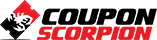# Free Statistics Tutorial – Least Squares Method: Theory and Implementation

A Hands-on Approach – Free Course

### What you’ll learn

• Theory of Least Squares Method
• Python Implementation of Least Squares Method
• MATLAB Implementation of Least Squares Method
• JavaScript Implementation of Least Squares Method

### Requirements

• Basic Mathematics
• Python Programming
• MATLAB Programming
• JavaScript Programming

### Description

In this tutorial firstly the mathematical foundations of a special case of Least Squares method has been reviewed. Then, using three programming languages, MATLAB, Python and JavaScript (using mathjs), the method has been implemented, from scratch

By the end of this course you will be able to know about the fundamental theory of least squares method and implementing that using Python, MATLAB and JavaScript programming languages .

Author(s): Yarpiz Team, Mostapha Kalami Heris

Deal Score+10
FREE 0